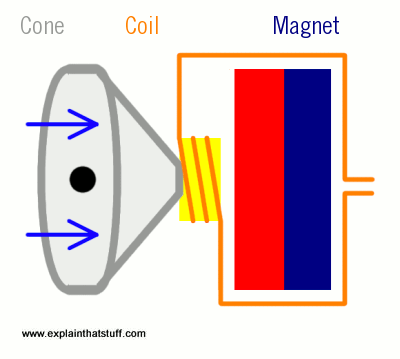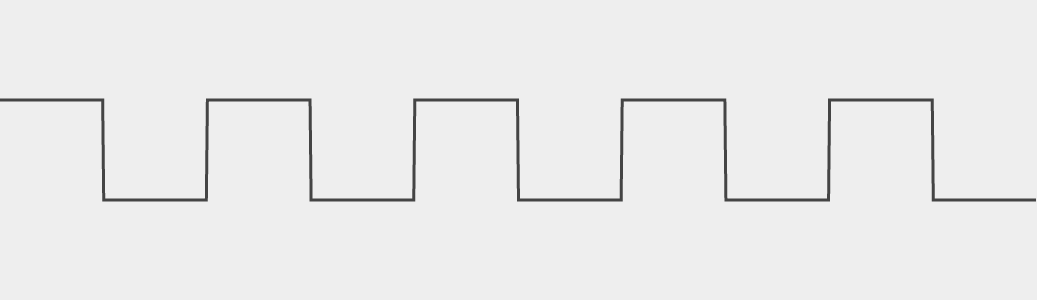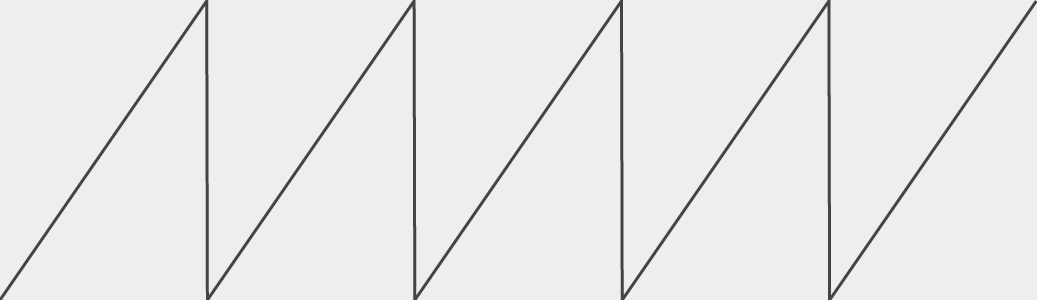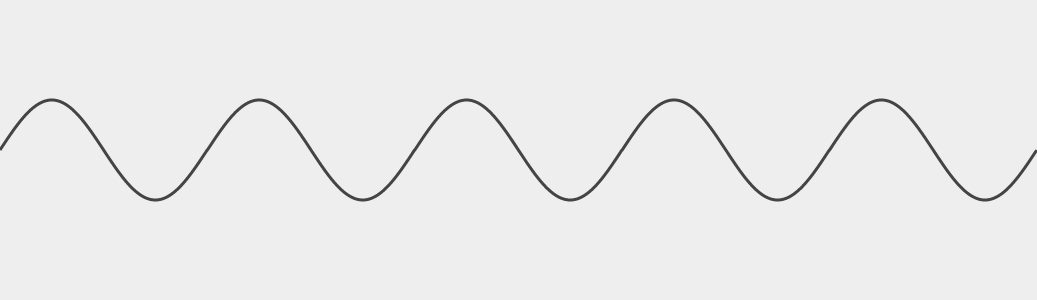# Programming Challenge #3 - Playing Sounds

Let's do more with these arrays that we can fill up. Did you know that all sound is made up of waves in the air? We can use these arrays of numbers to create waves in the air. First we should learn how sound on computers work!

All speakers are made out of a thing piece of material (sometimes called a 'cone') that vibrates back and forth to create air waves. Usually, they use a magnet that pulls on the membrane to move the cone.A computer creates sound by sending negative and positive numbers to the speaker. A negative number will suck the cone in and a positive number will push it out. Let's try using what we learned in the last lesson to make some noise using something called a 'triangle wave'.

```    var myArray = [];
for (var i = -150; i < 150; i++) {
myArray.push(i);
}
for (var i = 150; i > -150; i--) {
myArray.push(i);
}
draw(myArray);
```

You might notice that we are using `i++` instead of `i = i + 1` and `i--` instead of `i = i - 1`. They mean the same thing, it's just less typing. You can also do things like `i+=2` to add `2` to `i` or `i-=3` to subtract `3`.

Now let's copy that example code and run it to see what happens.

## Result

Looks like a triangle right? Well, let's put a couple of them together. Notice how we are using `repeat` to fill up the array 5 times. Also, instead of using `i--` to count down from 150, we `push(-i)` onto the array instead. Think about how that works.

```    var myArray = [];
for (var repeat = 0; repeat < 5; repeat++) {
for (var i = -150; i < 150; i++) {
myArray.push(i);
}
for (var i = -150; i < 150; i++) {
myArray.push(-i);
}
}
draw(myArray);
```

## Result

Cool, that is a triangle wave. Let's see what it sounds like!!

```    var myArray = [];
for (var repeat = 0; repeat < 5; repeat++) {
for (var i = -50; i < 50; i++) {
myArray.push(i);
}
for (var i = -50; i < 50; i++) {
myArray.push(-i);
}
}
draw(myArray);
play(myArray);
```

## Result

You can make it louder by increasing how positive and negative the numbers are. Notice that we are multiplying `i` by `5` when we do `push(i * 5)`.

```    var myArray = [];
for (var repeat = 0; repeat < 5; repeat++) {
for (var i = -50; i < 50; i++) {
myArray.push(i * 5);
}
for (var i = -50; i < 50; i++) {
myArray.push(-i * 5);
}
}
draw(myArray);
play(myArray);
```

Feel free to experiment with different values for `i * 5` to make it louder and quieter. For example, `i * 0.5` will make it quieter.

## Result

We can make it higher pitched by moving through the loop faster. Notice that we are doing `i += 2` now.

```    var myArray = [];
for (var repeat = 0; repeat < 5; repeat++) {
for (var i = -50; i < 50; i += 2) {
myArray.push(i);
}
for (var i = -50; i < 50; i += 2) {
myArray.push(-i);
}
}
draw(myArray);
play(myArray);
```

Feel free to experiment with different values instead of `i += 2`.

## Result

We can make it lower pitched by moving through the loop slower. Notice that we are doing `i += 0.5` now.

```    var myArray = [];
for (var repeat = 0; repeat < 5; repeat++) {
for (var i = -50; i < 50; i += 0.5) {
myArray.push(i);
}
for (var i = -50; i < 50; i += 0.5) {
myArray.push(-i);
}
}
draw(myArray);
play(myArray);
```

Feel free to experiment with different values instead `i += 0.5`.

# The First Challenge

You can make different sounds by changing the shape of the wave. This is something called a square wave.It is made with an array that looks like

`[ 50, 50,..a hundred 50's)..50, 50, -50, -50, -50,..(a hundred -50's)..-50, -50, 50, 50,..(another hundred 50's)..50,50...]`

Can you make one and see what it sounds like?

# The Second Challenge

There's another one called the sawtooth wave. It look like this.It is made with an array that looks like

`[ -50, -49, -48, -47, ... 47, 48, 49, 50, -50, -49, -48, -47, ... 47, 48, 49, 50, -50, -49 ...]`

Can you make that one?

# The Third Challenge

This one is going to be a bit different. Instead of trying to make a wave, what if we just make the speaker move around randomly. There is a function called `Math.random` that will create a random decimal from `0.000` to `1.000` for you. If you multiply it by `50` by doing `Math.random() * 50`, it will give you a number between 0 and 50.

Can you fill an array with 1000 random numbers and see what it looks and sounds like?

HINT: You can add a random number to an array using `myArray.push(Math.random() * 100)`

## Result

There's an extra fun bit to that one - if you hit Run over and over again, it will look and sound different because it's random!

# The Fourth Challenge

There is a special wave called a 'Sine' wave that is super smooth (and sounds smooth too!). It look like this:It is based on the `Math.sin` function and it takes decimal values and converts them into values from `-1.0` to `1.0`. It'll complete a full cycle of the numbers if you give it values from 0 to 2 x π.

Can you fill an array with a sine wave and play it?

HINTS: You can add the result of the sine function to an array by using `myArray.push(Math.sin(i))` and it might be useful to change `i` using small decimals like `0.01`. The sounds will be smoothest if you go from `0` to `2 * Math.PI`. And since `Math.sin(i)` only gives back numbers from `-1.0` to `1.0`, you might want to make it bigger somehow.